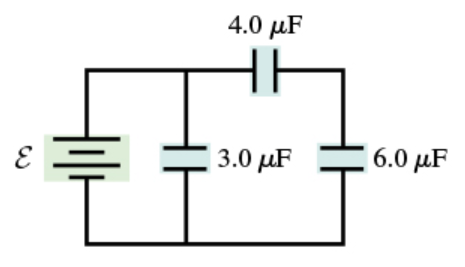# Problem: Consider the circuit shown below. Assume ε = 19 V.A. What is the charge on 3.0 μF capacitor?B. What is the charge on 4.0 μF capacitor?C. What is the charge on 6.0 μF capacitor?

###### FREE Expert Solution

Charge:

$\overline{){\mathbf{Q}}{\mathbf{=}}{\mathbf{C}}{\mathbf{V}}}$

Equivalent capacitance for two capacitors in series:

$\overline{){{\mathbf{C}}}_{\mathbf{e}\mathbf{q}}{\mathbf{=}}\frac{{\mathbf{C}}_{\mathbf{1}}{\mathbf{C}}_{\mathbf{2}}}{{\mathbf{C}}_{\mathbf{1}}\mathbf{+}{\mathbf{C}}_{\mathbf{2}}}}$

A.

Charge, Q = (3.0 × 10-6)(19) = 5.7 × 10-5 C

The charge on 3.0 μF capacitor is 5.7 × 10-5 C.

98% (451 ratings)###### Problem Details

Consider the circuit shown below. Assume ε = 19 V.A. What is the charge on 3.0 μF capacitor?

B. What is the charge on 4.0 μF capacitor?

C. What is the charge on 6.0 μF capacitor?